### 15.4.2.1 Completely integrable or nonholonomic?

Every control-affine system must be one or the other (not both) of the following:

1. Completely integrable: This means that the Pfaffian form (15.59) can be obtained by differentiatingequations of the form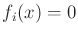with respect to time. This case was interpreted as being trapped on a surface in Section 13.1.3. An example of being trapped on a circle in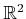was given in (13.22).
2. Nonholonomic: This means that the system is not completely integrable. In this case, it might even be possible to reach all of, even if the number of action variables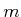is much smaller than, the dimension of.
In this context, the term holonomic is synonymous with completely integrable, and nonintegrable is synonymous with nonholonomic. The term nonholonomic is sometimes applied to non-Pfaffian constraints ; however, this will be avoided here, in accordance with mechanics literature .

The notion of integrability used here is quite different from that required for (14.1). In that case, the state transition equation needed to be integrable to obtain integral curves from any initial state. This was required for all systems considered in this book. By contrast, complete integrability implies that the system can be expressed without even using derivatives. This means that all integral curves can eventually be characterized by constraints that do not involve derivatives.

To help understand complete integrability, the notion of an integral curve will be generalized from one todimensions. A manifold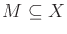is called an integral manifold of a set of Pfaffian constraints if at every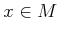, all vectors in the tangent space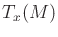satisfy the constraints. For a set of completely integrable Pfaffian constraints, a partition ofinto integral manifolds can be obtained by defining maximal integral manifolds from every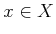. The resulting partition is called a foliation, and the maximal integral manifolds are called leaves .

Example 15..6 (A Foliation with Spherical Leaves)   As an example, suppose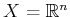and consider the Pfaffian constraint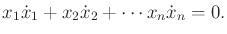(15.61)

This is completely integrable because it can be obtained by differentiating the equation of a sphere,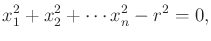(15.62)

with respect to time (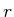is a constant). The particular sphere that is obtained via integration depends on an initial state. The foliation is the collection of all concentric spheres that are centered at the origin. For example, if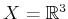, then a maximal integral manifold arises for each point of the form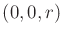. In each case, it is a sphere of radius. The foliation is generated by selecting every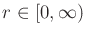.The task in this section is to determine whether a system is completely integrable. Imagine someone is playing a game with you. You are given an control-affine system and asked to determine whether it is completely integrable. The person playing the game with you can start with equations of the form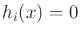and differentiate them to obtain Pfaffian constraints. These can then be converted into the parametric form to obtain the state transition equation (15.53). It is easy to construct challenging problems; however, it is very hard to solve them. The concepts in this section can be used to determine only whether it is possible to win such a game. The main tool will be the Frobenius theorem, which concludes whether a system is completely integrable. Unfortunately, the conclusion is obtained without producing the integrated constraints. Therefore, it is important to keep in mind that integrability'' does not mean that you can integrate it to obtain a nice form. This is a challenging problem of reverse engineering. On the other hand, it is easy to go in the other direction by differentiating the constraints to make a challenging game for someone else to play.

Steven M LaValle 2020-08-14